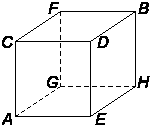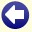## Solution to: Cube Creatures

We shall refer to the vertices of the cube with the following letters:We shall refer to the average time (in years) that a cube creature has still to live in a certain vertex with the same letter as the vertex itself.

If the cube creature arrives in point B, it dies. The average time that the animal has still to live in point B is therefore 0 years:

B = 0

If the cube creature is in point F, it can go three directions: to point B, C, or G. If it walks to point B, it has still 1 year to live. If it walks to point C, it has still to live 1 year plus the average time that it has still to live in point C. If it walks to point G, it has still to live 1 year plus the average time that it has still to live in point G. Each of the three directions has equal probability to be chosen, so the probability is 1/3 for each direction. The average time that the creature has still to live if it is in point F zit is therefore:

F = 1/3×1 + 1/3×(1+C) + 1/3×(1+G) = 1/3C + 1/3G + 1

In the same way, we can calculate the time to live for all other vertices:

A = 1/3C + 1/3E + 1/3G + 1
C = 1/3A + 1/3D + 1/3F + 1
D = 1/3C + 1/3E + 1
E = 1/3A + 1/3D + 1/3H + 1
G = 1/3A + 1/3F + 1/3H + 1
H = 1/3E + 1/3G + 1

A = 1/3C + 1/3E + 1/3G + 1

and substitute the formulas for C, E and G:

A = 1/3×(1/3A + 1/3D + 1/3F + 1) + 1/3×(1/3A + 1/3D + 1/3H + 1) + 1/3×(1/3A + 1/3F + 1/3H + 1) + 1

which can be rewritten to:

A = 1/3A + 2/9D + 2/9F + 2/9H + 2.

Now we substitute the formulas for D, F and H, which results in:

A = 1/3A + 4/27C + 4/27E + 4/27G + 4/9 + 22/9.

Because A = 1/3C + 1/3E + 1/3G + 1, it also holds that 4/9A = 4/27C + 4/27E + 4/27G + 4/9. If we fill this in, we get:

A = 1/3A + 4/9A + 22/9

from which follows that A=10. Conclusion: cube creatures live 10 years on average.Back to the puzzle
This website uses cookies. By further use of this website, or by clicking on 'Continue', you give permission for the use of cookies. If you want more information, look at our cookie policy.